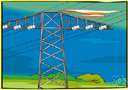# potential divider

Also found in: Thesaurus, Encyclopedia, Wikipedia.

## potential divider

n
(Electronics) a tapped or variable resistor or a chain of fixed resistors in series, connected across a source of voltage and used to obtain a desired fraction of the total voltage. Also called: voltage divider
ThesaurusAntonymsRelated WordsSynonymsLegend:
 Noun 1potential divider - resistors connected in series across a voltage source; used to obtain a desired fraction of the voltagevoltage dividerpotentiometer, pot - a resistor with three terminals, the third being an adjustable center terminal; used to adjust voltages in radios and TV setsresistor, resistance - an electrical device that resists the flow of electrical current
Mentioned in ?
References in periodicals archive ?
The discharge gap voltage was measured using the North Star High Voltage compensated capacitance-ohm potential divider (PD) of a PVM-12 type with the dividing coefficient of 1:1000, bandwidth of 80 MHz and the maximum pulse voltage of 32 kV.
The potential divider output is connected to amplifier section.
Tenders are invited for Supply of High Voltage Potential Divider

Site: Follow: Share:
Open / Close